Suppose you wish to construct a motor that produces a maximum torque whose magnitude is 1.7 × 10-2 N·m. The coil of the motor has an area of

Question

Suppose you wish to construct a motor that produces a maximum torque whose magnitude is 1.7 × 10-2 N·m. The coil of the motor has an area of 9.0 × 10-4 m2, consists of N turns, and contains a current of 1.1 A. The coil is placed in a uniform magnetic field of magnitude 0.20 T. What must N be?

in progress 0
5 months 2021-08-29T12:30:30+00:00 1 Answers 5 views 0

The number of turns in the coil is 86.

Explanation:

Given that,

The magnitude of maximum torque produced in the motor,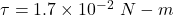Area of the coil,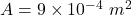Current in the coil, I = 1.1 A

Magnetic field in the coil, B = 0.2 T

We need to find the value of N i.e. number of turns in the coil. The magnitude of torque attained in the coil is given by :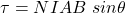Here,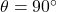(maximum)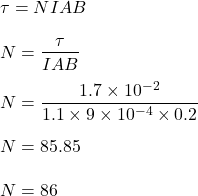So, the number of turns in the coil is 86. Hence, this is the required solution.